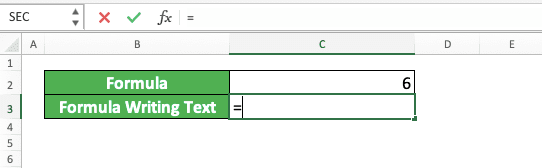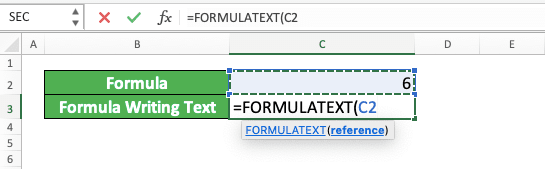How to Use the FORMULATEXT Formula in Excel: Functions, Examples, and Writing Steps - Compute Expert

# How to Use the FORMULATEXT Formula in Excel: Functions, Examples, and Writing Steps

Home >> Excel Tutorials from Compute Expert >> Excel Formulas List >> How to Use the FORMULATEXT Formula in Excel: Functions, Examples, and Writing Steps

In this tutorial, you will learn how to use the FORMULATEXT formula in excel completely.

When working in excel, we might sometimes want to show the formula we have written in our cell. One of the methods you can use to do that is by using FORMULATEXT.

Want to know more about this FORMULATEXT function and master the way to use it properly in excel? Read this tutorial until its last part!

Disclaimer: This post may contain affiliate links from which we earn commission from qualifying purchases/actions at no additional cost for you. Learn more

## What is the FORMULATEXT Formula in Excel?

FORMULATEXT is an excel function that helps you to get the text of a formula writing in a cell.

## FORMULATEXT Function in Excel

You can use FORMULATEXT to get the text of a formula you write in a cell.

## FORMULATEXT Result

The FORMULATEXT result is a text which contains the formula writing in a cell you input into it.

## Excel Version from Which We Can Start Using FORMULATEXT

We can start using FORMULATEXT in excel since excel 2013.

## The Way to Write It and Its Input

Here is the way to write FORMULATEXT in excel.

= FORMULATEXT ( cell_with_formula )

When writing FORMULATEXT, you only need to input the coordinate of the cell you want to get your formula writing from.

## Example of Its Usage and Result

Here is an implementation example of FORMULATEXT in excel.As you can see there, we can get the formula writing in our cell by using FORMULATEXT. Just input the cell containing the formula to FORMULATEXT, press enter, and we will immediately get our formula writing!

If you input a cell that doesn’t contain a formula, FORMULATEXT will produce a #N/A error (as you can see in the last row of the example).

## Writing Steps

After we have discussed the FORMULATEXT writing form, input, and implementation example, now let’s discuss its writing steps. As FORMULATEXT only needs one simple input in its writing (the cell which contains the formula writing we want to get), its writing steps are easy to understand.

1. Type an equal sign ( = ) in the cell where you want to place your formula writing text2. Type FORMULATEXT (can be with large and small letters) and an open bracket sign after =3. Input the cell coordinate that contains the formula writing you want to get4. Type a close bracket sign5. Press Enter
6. Done!## Calculate the Length of a Formula: LEN FORMULATEXT

Through FORMULATEXT, you can also know the length of your formula writing in a cell. Just combine it with LEN to get that information for you.

Here is the general writing form of the LEN and FORMULATEXT combination in excel.

= LEN ( FORMULATEXT ( cell_with_formula ) )

Simple, isn’t it? Just write your FORMULATEXT in LEN and input the cell you want to get the formula length of to the FORMULATEXT. By doing that, you will immediately get the formula writing length inside the cell you want!

Here is its implementation example in excel.## Get the Formula Writing or Another Result: IF ISFORMULA FORMULATEXT

If you want to check first whether a cell contains a formula before you use FORMULATEXT, do this. Combine IF and ISFORMULA with your FORMULATEXT function to get what you want.

By doing the combination of those functions, you can produce another result if your cell doesn’t contain a formula. Here is the general writing form of the three functions combination in excel.

= IF ( ISFORMULA ( cell_with_formula ) , FORMULATEXT ( cell_with_formula ) , another_result )

In this formula writing process, ISFORMULA will check first whether your cell contains formula writing. If it is, then ISFORMULA will produce TRUE and your IF TRUE result, FORMULATEXT, will run. Because of that, you will get a formula writing text if your cell indeed contains a formula.

On the other hand, if it doesn’t contain a formula, IF will produce its FALSE result. You can determine what IF will produce in this situation with your IF FALSE result input.

Here is an implementation example of the functions combination concept in excel.In the example, we get the formula writing of our cell if it contains a formula. If it doesn’t contain a formula, we get a “Not a Formula” text instead. That is because we combine IF, ISFORMULA, and FORMULATEXT as discussed and put “Not a Formula” in our IF FALSE

## Exercise

After we have learned how to use FORMULATEXT in excel properly, let’s do an exercise to deepen our understanding!

### Instructions

Give your answer by using the help of FORMULATEXT in the appropriate gray-colored cell according to the question number!
1. What is the text of the formula writing on the left?
2. What is the length of the formula on the left?
3. Does the cell on the left contain a formula? Get the formula writing text if it does and answer “No, It Does Not” if it doesn’t!

• You can input a cell that contains a formula writing with up to 8192 characters in length to FORMULATEXT
• If you want to show all your formula writings directly in their cell, you can just press Control + ` to do that

Related tutorials you should read too:

Get updated excel info from Compute Expert by registering your email. It's free!